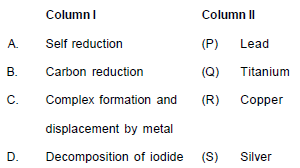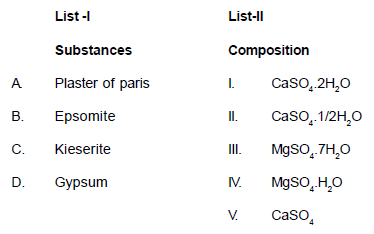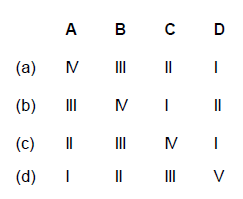Courses

# Extraction Of Metals (Chapter Test - Non-Medical)

## 20 Questions MCQ Test Chemistry for IIT JEE (Main & Advanced) | Extraction Of Metals (Chapter Test - Non-Medical)

Description
This mock test of Extraction Of Metals (Chapter Test - Non-Medical) for Class 12 helps you for every Class 12 entrance exam. This contains 20 Multiple Choice Questions for Class 12 Extraction Of Metals (Chapter Test - Non-Medical) (mcq) to study with solutions a complete question bank. The solved questions answers in this Extraction Of Metals (Chapter Test - Non-Medical) quiz give you a good mix of easy questions and tough questions. Class 12 students definitely take this Extraction Of Metals (Chapter Test - Non-Medical) exercise for a better result in the exam. You can find other Extraction Of Metals (Chapter Test - Non-Medical) extra questions, long questions & short questions for Class 12 on EduRev as well by searching above.
QUESTION: 1

Solution:
QUESTION: 2

Solution:
QUESTION: 3

### Which is known as blister copper?

Solution:
QUESTION: 4

A common metal used as reductant for the extraction of metals from their oxides is

Solution:
QUESTION: 5

Match the extraction process listed in column I with metals listed in column II.Solution:
QUESTION: 6

The method of Zone refining of metals is based on the principal of

Solution:
QUESTION: 7

Which represents calcination ?

Solution:
QUESTION: 8

All ores are minerals, while all minerals are not ores, because ....

Solution:
QUESTION: 9

The metal extracted by leaching of a cyanide is

I. Silver                II. Sodium
III. Gold               IV. Copper

Solution:
QUESTION: 10

Choose the correct code regarding roasting process.
I. It is the process of heating ore in air to obtain the oxide.
II. It is an exothermic process.
III. It is used for hydrated oxide and oxygen ore.
IV. It is used after the concentration of ore.

Solution:
QUESTION: 11

Extraction of group 1 and 2 metals is usually done by

Solution:
QUESTION: 12

Heating mixture of Cu2O and Cu2S will give

Solution:
QUESTION: 13

Which of the following processes involves smelting ?

Solution:
QUESTION: 14

Which of the following processes does not involve oxidation of iron ?

Solution:
QUESTION: 15

Which of the following statements is correct regarding self reduction ?

Solution:
QUESTION: 16

Match List -I with List -II for the composition of substances and select the correct answer using the code given below the listsSolution:
QUESTION: 17

Which of the following curves assures that the metal obtained by carbon reduction is in the vapour state ?

Solution:
QUESTION: 18

Which of the following activities is not related to poling ?

Solution:
QUESTION: 19

(a) If both assertion and reason are correct and reason is the correct explanation of assertion.
(b) If both assertion and reason are correct but reason is not correct explanation of assertion.
(c) If assertion is correct and reason is incorrect.
(d) If assertion is incorrect and reason is correct.

Q.

Assertion : Ag and Au are extracted by leaching their ores with a dilute solution of NaCN.
Reason : Impurities associated with these ores dissolve in NaCN

Solution:
QUESTION: 20

(a) If both assertion and reason are correct and reason is the correct explanation of assertion.
(b) If both assertion and reason are correct but reason is not correct explanation of assertion.
(c) If assertion is correct and reason is incorrect.
(d) If assertion is incorrect and reason is correct.

Q.

Assertion : During calcination, the ore is heated well below its melting point in the limited supply of oxygen.

Reason : The process of calcination is carried out for sulphide ores.

Solution:

The Correct Reason: Heating of the ore below its melting point, in limites supply of oxygen, ensures removal of volatile impurities, water from hydrated ore, CO2CO2from carbonate pre.